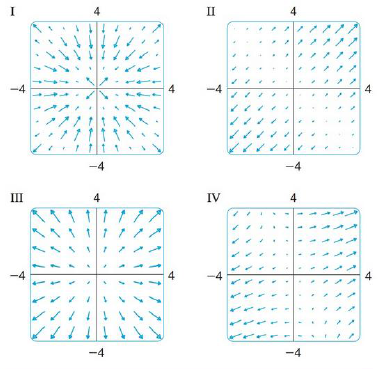Chapter 16.1, Problem 29E

Chapter
Section
Textbook Problem

Match the functions f with the plots of their gradient vector fields labeled I-IV. Give reasons for your choices.29. f(x, y) = x2 + y2To determine

To match: The function f(x,y)=x2+y2 with the plots labelled as I-IV.

Explanation

Given data:

f(x,y)=x2+y2

Formula used:

Write the expression for gradient vector field of two dimensional vector.

f(x,y)=fxi+fyj (1)

Write the required differentiation formulae with respect to x as follows.

x(x2)=2xx(y2)=0

Write the required differentiation formulae with respect to y as follows.

y(x2)=0y(y2)=2y

Differentiate the term x2+y2 with respect to x .

x(x2+y2)=x(x2)+x(y2)=2x+0=2x

Differentiate the term x2+y2 with respect to y

Still sussing out bartleby?

Check out a sample textbook solution.

See a sample solution

The Solution to Your Study Problems

Bartleby provides explanations to thousands of textbook problems written by our experts, many with advanced degrees!

Get Started

Factoring Completely Factor the expression completely. 115. 8x3 125

Precalculus: Mathematics for Calculus (Standalone Book)

Calculate y'. 50. y=sin2(cossinx)

Single Variable Calculus: Early Transcendentals, Volume I

A sample of n = 9 scores has X = 108. What is the sample mean?

Essentials of Statistics for The Behavioral Sciences (MindTap Course List)

Year 2009 2010 2011 Sales, y 887 1028 1251 a. Plot the Cyber Monday sales (y) versus the year (t), where ...

Applied Calculus for the Managerial, Life, and Social Sciences: A Brief Approach

Compute C8,8.

Understanding Basic Statistics